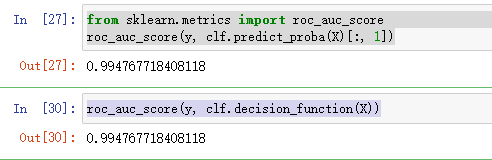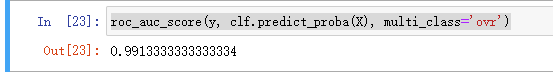• roc_auc_score
千次阅读
2021-01-08 22:24:59

### 引言

from sklearn.metrics import roc_auc_score

roc_auc_score(y_true, y_score, *, average='macro', sample_weight=None,
max_fpr=None, multi_class='raise', labels=None)
计算曲线ROC的面积

- Parameters(参数)
y_true : array-like of shape (n_samples,) or (n_samples, n_classes)
真实数据
二分类和多分类需要带有shape (n_samples)的标签，而多标签情况需要带有shape (n_samples, n_classes)
的二进制标签。

y_score : array-like of shape (n_samples,) or (n_samples, n_classes)
预测结果数据
1.在二分类的情况下，它对应于形状数组（n_samples，），可以提供概率估计和非阈值决策值
概率估计值对应于具有更大标签的类别的概率，即estimator.classes_ ，
因此是estimator.predict_proba（X，y）[:, 1]。
决策值对应于estimator.decision_function（X，y）的输出。
2.在多分类情况下，它对应于由predict_proba方法提供的概率估计的形状数组（n_samples，n_classes）
每个sample概率估计值为1；此外，每一个sample的概率估计值的顺序必须与y_true中标签的顺序相对应。
3.在多标签情况下，它对应于一个形状数组（n_samples，n_classes）。
概率估计由predict_proba方法提供，非阈值决策值由decision_function方法提供。

average : {‘micro’, ‘macro’, ‘samples’, ‘weighted’} or None, default=’macro’
当y_true是二进制时，这个参数将被忽略
'macro':简单地计算 binary metrics （二分指标）的平均值，赋予每个类别相同的权重
'micro':给每个 sample-class pair （样本类对）对 overall metric （总体指数）
（sample-class 权重的结果除外） 等同的贡献。除了对每个类别的 metric 进行求和之外，这
个总和构成每个类别度量的 dividends （除数）和 divisors （除数）计算一个整体商。 在
multilabel settings （多标签设置）中，Micro-averaging 可能是优先选择的，包括要忽略
majority class （多数类）的 multiclass classification （多类分类）
'weighted': 通过计算其在真实数据样本中的存在来对每个类的 score 进行加权的 binary metrics （二分指标）
的平均值来计算类不平衡。
'samples':仅适用于多标签问题。它不计算每个类别的 measure，而是计算评估数据中的每个样本
的真实和预测类别的 metric （指标），并返回 (sample_weight-weighted) 加权平均。
sample_weight :array-like of shape (n_samples,), default=None
样品权重

sample_weight : array-like of shape (n_samples,), default=None
如果不为None，则返回范围为[0，max_fpr]的标准化部分AUC
对于多分类情况，max_fpr应该等于None或1.0

multi_class:{‘raise’, ‘ovr’, ‘ovo’}, default=’raise’
仅用于多分类，默认值会引发错误，因此必须显式传递'ovr'或'ovo'
'ovr':一对多
'ovo':一对一
这两个概念想了解的参考为2.逻辑回归部分

labels : array-like of shape (n_classes,), default=None
仅用于多分类，标签列表索引了y_score中的类，如果为None，则使用y_true中标签的数字或字典顺序

- 返回
AUC值



### 官方案例

二分类情况

>>> from sklearn.datasets import load_breast_cancer
>>> from sklearn.linear_model import LogisticRegression
>>> from sklearn.metrics import roc_auc_score
>>> clf = LogisticRegression(solver="liblinear", random_state=0).fit(X, y)
>>> roc_auc_score(y, clf.predict_proba(X)[:, 1])
0.99...
>>> roc_auc_score(y, clf.decision_function(X))
0.99...


多分类情况

>>> from sklearn.datasets import load_iris
>>> clf = LogisticRegression(solver="liblinear").fit(X, y)
>>> roc_auc_score(y, clf.predict_proba(X), multi_class='ovr')
0.99...


多标签情况

>>> from sklearn.datasets import make_multilabel_classification
>>> from sklearn.multioutput import MultiOutputClassifier
>>> X, y = make_multilabel_classification(random_state=0)
>>> clf = MultiOutputClassifier(clf).fit(X, y)
>>> # get a list of n_output containing probability arrays of shape
>>> # (n_samples, n_classes)
>>> y_pred = clf.predict_proba(X)
>>> # extract the positive columns for each output
>>> y_pred = np.transpose([pred[:, 1] for pred in y_pred])
>>> roc_auc_score(y, y_pred, average=None)
array([0.82..., 0.86..., 0.94..., 0.85... , 0.94...])
>>> from sklearn.linear_model import RidgeClassifierCV
>>> clf = RidgeClassifierCV().fit(X, y)
>>> roc_auc_score(y, clf.decision_function(X), average=None)
array([0.81..., 0.84... , 0.93..., 0.87..., 0.94...])


### 案例

import numpy as np
# 模型评估
from sklearn import metrics

if __name__ == '__main__':
y = np.array([0, 0, 1, 1])
y_pred = np.array([0.1, 0.5, 0.3, 0.8])

# 返回三个数组结果分别是fpr(假正率),tpr(召回率),threshold(阈值)
# 参数为真实结果数据、预测结果数据（可以是标签数据也可以是概率值）
fpr, tpr, threshold = metrics.roc_curve(y, y_pred)
# 计算AUC的值
auc = metrics.auc(fpr, tpr)
print(auc)
print(metrics.roc_auc_score(y, y_pred))

0.75
0.75


当碰到多分类情况时，可以使用one-hot编码，在进行ravel()展平操作，来使用

auc = roc_auc_score(y_one_hot.ravel(), y_score.ravel())


如果对您有帮助，麻烦点赞关注，这真的对我很重要！！！如果需要互关，请评论留言！更多相关内容
• roc_auc_score(y_true, y_score, *, average='macro', sample_weight=None, max_fpr=None, multi_class='raise', labels=None) 计算曲线ROC的面积 - Parameters(参数) y_true : array-like of shape (n_samples,) ...

参数说明

from sklearn.metrics import roc_auc_score

roc_auc_score(y_true, y_score, *, average='macro', sample_weight=None,
max_fpr=None, multi_class='raise', labels=None)
计算曲线ROC的面积

- Parameters(参数)
y_true : array-like of shape (n_samples,) or (n_samples, n_classes)
真实数据
二分类和多分类需要带有shape (n_samples)的标签，而多标签情况需要带有shape (n_samples, n_classes)
的二进制标签。

y_score : array-like of shape (n_samples,) or (n_samples, n_classes)
预测结果数据
1.在二分类的情况下，它对应于形状数组（n_samples，），可以提供概率估计和非阈值决策值
概率估计值对应于具有更大标签的类别的概率，即estimator.classes_ ，
因此是estimator.predict_proba（X，y）[:, 1]。
决策值对应于estimator.decision_function（X，y）的输出。
2.在多分类情况下，它对应于由predict_proba方法提供的概率估计的形状数组（n_samples，n_classes）
每个sample概率估计值为1；此外，每一个sample的概率估计值的顺序必须与y_true中标签的顺序相对应。
3.在多标签情况下，它对应于一个形状数组（n_samples，n_classes）。
概率估计由predict_proba方法提供，非阈值决策值由decision_function方法提供。

average : {‘micro’, ‘macro’, ‘samples’, ‘weighted’} or None, default=’macro’
当y_true是二进制时，这个参数将被忽略
'macro':简单地计算 binary metrics （二分指标）的平均值，赋予每个类别相同的权重
'micro':给每个 sample-class pair （样本类对）对 overall metric （总体指数）
（sample-class 权重的结果除外） 等同的贡献。除了对每个类别的 metric 进行求和之外，这
个总和构成每个类别度量的 dividends （除数）和 divisors （除数）计算一个整体商。 在
multilabel settings （多标签设置）中，Micro-averaging 可能是优先选择的，包括要忽略
majority class （多数类）的 multiclass classification （多类分类）
'weighted': 通过计算其在真实数据样本中的存在来对每个类的 score 进行加权的 binary metrics （二分指标）
的平均值来计算类不平衡。
'samples':仅适用于多标签问题。它不计算每个类别的 measure，而是计算评估数据中的每个样本
的真实和预测类别的 metric （指标），并返回 (sample_weight-weighted) 加权平均。
sample_weight :array-like of shape (n_samples,), default=None
样品权重

sample_weight : array-like of shape (n_samples,), default=None
如果不为None，则返回范围为[0，max_fpr]的标准化部分AUC
对于多分类情况，max_fpr应该等于None或1.0

multi_class:{‘raise’, ‘ovr’, ‘ovo’}, default=’raise’
仅用于多分类，默认值会引发错误，因此必须显式传递'ovr'或'ovo'
'ovr':一对多
'ovo':一对一
这两个概念想了解的参考为2.逻辑回归部分

labels : array-like of shape (n_classes,), default=None
仅用于多分类，标签列表索引了y_score中的类，如果为None，则使用y_true中标签的数字或字典顺序

- 返回
AUC值

获取auc的方式

二分类：

from sklearn.datasets import load_breast_cancer
from sklearn.linear_model import LogisticRegression
from sklearn.metrics import roc_auc_score
clf = LogisticRegression(solver="liblinear", random_state=0).fit(X, y)

from sklearn.metrics import roc_auc_score
#方式一
roc_auc_score(y, clf.predict_proba(X)[:, 1])
#方式二
roc_auc_score(y, clf.decision_function(X))多分类：

from sklearn.datasets import load_iris

clf = LogisticRegression(solver="liblinear").fit(X, y)
clf.predict_proba(X)

from sklearn.metrics import roc_auc_score
#multi_class='ovr'
roc_auc_score(y, clf.predict_proba(X), multi_class='ovr')https://blog.csdn.net/weixin_46649052/article/details/112384745

展开全文• predict和predict_proba带入roc_auc_score结果不同 我们都知道AUC表示ROC曲线下的面积，下面两种计算格式（二分类）： # 带入预测的概率进行计算 roc1=roc_auc_score(y_test, model.predict_proba(x_test)[:, 1]) # ...

## predict和predict_proba带入roc_auc_score结果不同

我们都知道AUC表示ROC曲线下的面积，下面两种计算格式（二分类）：

# 带入预测的概率进行计算
roc1=roc_auc_score(y_test, model.predict_proba(x_test)[:, 1])
# 带入预测的分类进行计算
roc2=roc_auc_score(y_test, model.predict(x_test))


结果是roc2小于roc1

### 为什么呢？

如图，ROC是通过将不同threshold的x轴（FPR）和y轴（TPR）的点连接而成的曲线， AUC就是ROC下的面积。

threshold：划分预测分类的分界，一般大于0.5就认为是target，但是没规定必须是0.5，也可以是其他值predict_proba得到的是预测的概率，不同的threshold会有不同的TPR和FPR，可以得到上图圆滑的曲线

predict则不同，输出结果的时候已经固定了threshold，默认为0.5，所以它的roc曲线呈现以下格式，一种折线的形式如果是相同模型，同时画出这两条曲线，折线会在曲线的下方，这也解释了为什么前面计算的roc结果不同，同时roc2小于roc1的原因，因为曲线下的面积不同，同时曲线下的面积大于折线的面积

### 参考

sklearn学习：为什么roc_auc_score()和auc()有不同的结果？：https://www.programminghunter.com/article/4582657422/

展开全文sklearn 机器学习 python
• sklearn.metrics.classification_report(y_true, y_pred, labels=None, target_names=...sklearn.metrics.roc_auc_score(y_true, y_score, *, average='macro', sample_weight=None, max_fpr=None, multi_class='rai.

sklearn.metrics.classification_report(y_true, y_pred, labels=None, target_names=None, sample_weight=None, digits=2, output_dict=False)

sklearn.metrics.roc_auc_score(y_true, y_score, *, average='macro', sample_weight=None, max_fpr=None, multi_class='raise', labels=None)[source])

上面的y_pred和y_score完全不一样，

y_pred:  预测值的标签，类标签的列表。比如[0, 0, 1, 0, 1]

y_score：目标分数。形状(n_samples，)或(n_samples, n_classes)。二分类情况形状(n_samples，1)，“分数必须是具有较大标签的类的分数”，通俗点理解:模型打分的第二列。举个例子:模型输入的得分是一个数组[0.98361117 0.01638886]，索引是其类别，这里“较大标签类的分数”，指的是索引为1的分数：0.01638886，也就是正例的预测得分。

参考

展开全文sklearn 机器学习 python
• roc_auc_score则是不需要求这两个值，直接将预测概率值和label输入到函数中便可以求得auc值，省略了求这两个值的步骤，由函数本身代求。 这是我找很久的解释博客，才弄明白。 希望能帮助到大家。 ...python 机器学习
• sklearn中使用roc_auc_score()函数计算auc。 group true得是{0,1}n\{0,1\}^n{0,1}n, pred 理论上得是每个元素都是概率。用于多标签分类的话是得用sigmoid 激活后的（0，1）之间的概率值。 但是sklearn是可以直接没有...sklearn python
• sklearn：aucroc_curve、roc_auc_score_NockinOnHeavensDoor的博客-CSDN博客 切记： 1。 train的时候，若出现valuerror，只出现一个class在y_true的情况时，可通过提高batchsize的大小解决，这种情况的发生是...sklearn 分类 机器学习
•   了解一个函数首先看这个函数的功能，也就是这个函数的参数有哪些，这个函数的返回值是哪些。...accuracy_score（）函数 1、函数功能 分类正确率分数，函数返回一个分数，这个分数或是正确的比.python 机器学习 人工智能
• 最早发现这个问题是发觉输入roc_auc_score（xtest，prediction）输出的auc与plot_auc的值相差甚远，查资料之后发现关键在于第二个参数应该输入模型的输出概率值而非预测值，这里是个小坑 ，因为其他如acc pre f1...sklearn python 机器学习
• 二分类，sklearn包roc_curve计算曲线下面积 // 分割结果，单张图片AUC计算 ... from sklearn.metrics import roc_curve, auc out_s = out_s.squeeze(0).detach().numpy() gt_s = gt_s.squeeze(0).cpu().numpy().assklearn 分类 机器学习
• AUC并不总是ROC曲线下的面积 . 曲线下面积是 some 曲线下的(抽象)区域，因此它比AUROC...请参阅 roc_auc_score 的sklearn源：def roc_auc_score(y_true, y_score, average="macro", sample_weight=None):# docstring...
• ## sklearn：auc、roc_curve、roc_auc_score

万次阅读 多人点赞 2018-10-25 19:23:04
sklearn.metrics.auc 作用：计算AUC(Area Under the Curve) metrics.roc_curve 作用：计算 ROC(Receiver operating characteristic) 注意: this implementation is restricted to the binary classification task ...
• from sklearn.metrics import roc_auc_score y_true = [0, 0, 1, 1, 1] y_score = [0.1, 0.2, 0.7, 0.8, 0.9] print(roc_auc_score(y_true, y_score)) y_score = [0.7, 0.8, 0.9, 0.1, 0.2] print(roc_auc_score(y...
• AUC并不总是ROC曲线下的面积.曲线下面积是某个曲线下的(抽象)区域,...请参阅sklearn source for roc_auc_score：def roc_auc_score(y_true, y_score, average="macro", sample_weight=None):# docstring def _bin...
• import numpy as np from sklearn import metrics import matplotlib.pyplot as plt ...fpr,tpr,thresholds=metrics.roc_curve(label,scores) print('FPR:',fpr) print('TPR:',tpr) print('thresholds:',threshojson vscode
• （1）曲线与FP_rate轴围成的面积（记作AUC）越大，说明性能越好，即图上L2曲线对应的性能优于曲线L1对应的性能。即：曲线越靠近A点（左上方）性能越好，曲线越靠近B点（右下方）曲线性能越差。 （2）A点是最完美的...算法 python
• 首先，你的数据不管是库自带的如： from sklearn.datasets import load_breast_cancer...X = data.drop("score", axis=1).values Y = data["score"].values 都要注意保证你的数据都是numpy类型的 对于二分类 直接用 Y
• 写在前面： sklearn计算auc(一):使用sklearn.metrics.roc_auc_score()计算二分类的auc 1.sklearn.metrics.roc_auc_score()计算多分类auc的用法 2.例子python
• 首先，roc_auc_score函数需要具有相同形状的输入参数。sklearn.metrics.roc_auc_score(y_true, y_score, average=’macro’, sample_weight=None)Note: this implementation is restricted to the binary ...
• def plot_roc_curve(y_true, y_score): """ y_true:真实值 y_score：预测概率。注意：不要传入预测label！！！ """ from sklearn.metrics import roc_curve import matplotlib.pyplot as plt fpr,tpr,...
• 1、sklearn.model_selection.cross_val_score(estimator,X,y=None,groups=None,scoring=None,cv=None,n_jobs=None,verbose=0,fit_params=None,pre_dispatch='2*n_jobs',error_score=nan) （1）cv参数定义交叉验证...机器学习
• sklearn.metrics.roc_auc_score(y_true, y_score, *, average='macro', sample_weight=None, max_fpr=None, multi_class='raise', labels=None) 二分类 y_true：样本的真实标签，形状（样本数，） y_score：预测为1...
• AUC原理可看：https://editor.csdn.net/md/?articleId=104838433 >>> import numpy as np >>> from sklearn import metrics >>> y = np.array([1, 1, 2, 2]) >>> scores = np....
• 关于运行roc_auc_score(y_true, y_scores)报错：ValueError: multiclass format is not supported，原因在于ROC只能用于二分类问题，所以，对于多分类问题，我们不用ROC曲线去评估，可以选用accuracy_score进行评估 ...
• ROC（Receiver Operating Characteristic）曲线和AUC常被用来评价一个二值分类器（binary classifier）的优劣。 AUC（Area Under Curve）被定义为ROC曲线下的面积，显然这个面积的数值不会大于1。又由于ROC曲线一般...sklearn
• 1、accuracy_score 分类准确率分数是指所有分类正确的百分比。分类准确率这一衡量分类器的标准比较容易理解，但是它不能告诉你响应值的潜在分布，并且它也不能告诉你分类器犯错的类型。sklearn.metrics.accuracy_......